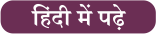Latest SSC jobs   »   Triangles: Notes and Questions

# Triangles: Notes and Questions

Mathematics is one of the most important that is asked in the government recruitment exams. Generally, there are questions asked related to basic concepts and formulas of trigonometry. To let you make the most of the Mathematics section, we are providing important notes related to triangle. Also, many central and state exams are nearby with bunches of posts for the interested candidates in which Mathematics is a major part. We have covered important notes and questions focusing on these prestigious exams. We wish you all the best of luck to come over the fear of the Mathematics section.

### Triangle

1). Centroid : [Intersecting Point of Medians ]/ केन्द्रक: माध्य का अंतर्बिंदु
2). Incenter → [Intersecting Point of Internal angle bisector] / अन्तः केन्द्र → [आंतरिक कोण द्विभाजक का प्रतिछेदन बिंदु ]
3).Circumcenter: → [Intersecting point of Perpendicular bisector] / परिकेंद्र :  [लम्बवत द्विभाजक का प्रतिछेदन बिंदु]
4). Orthocenter: → [Intersecting Point of Altitudes] / लंब केंद्र: → [ऊंचाई का प्रतिछेदन बिंदु]
Important Points:
(a)  Orthocenter of right angled triangle ⇒ at right angled vertex(समकोण त्रिभुज का लांब केंद्र ⇒   समकोण के शीर्ष पर )
(b)  Circumcenter of right angled triangle ⇒ Mid-point of Hypotenuse / समकोण का परिकेंद्र ⇒ मध्य बिंदु का कर्ण
(c)  Distance b/w incenter & circumcenter of a triangle / एक त्रिकोण के आतंरिक और परित्रिज्या के मध्य की दूरी
(d)  In Equilateral triangle / समबाहु त्रिभुज में,

### Questions

Q. Find the incentre of the triangle the coordinates of whose vertices are given by A(x1, y1), B(x2, y2), C(x3, y3).

Solution:

By geometry, we know that BD/DC = AB/AC (since AD bisects ÐA).
The lengths of the sides AB, BC and AC are c, a and b respectively, then BD/DC = AB/AC = c/b.
Coordinates of D are (bx2+cx3/b+c, by2+cy3/b+c)
IB bisects DB. Hence ID/IA = BD/BA = (ac/b+c)/c = a/c+b.
Let the coordinates of I be (x, y).
Then x = ax1+bx2+cx3/a+b+c, y = ay1+by2+cy3/a+b+c.

Q. If (0, 1), (1, 1) and (1, 0) are middle points of the sides of a triangle, find its incentre.

Solution:

Let A(x1, y1), B(x2, y2) and C(x3, y3)be teh vertices of a triangle.
x1 + x2 = 0, x2 + x= 0, x3 + x1 = 0
y1 + y2 = 0, y2 + y3 = 0, y3 + y1 = 0.
Solving these equations, we get A(0, 0), B(0, 2) and C(2, 0).
Now, a = BC = 2√ 2, b = CA = 2 and c = AB = 2.
Thus, incentre of the triangle ABC is (2-√ 2, 2-√ 2).

Q. If midpoints of the sides of a triangle are (0, 4), (6, 4) and (6, 0), then find the vertices of triangle, centroid and circumcentre of triangle.Solution:

Let points A (x1, y1), B (x2, y2) and C (x3, y3) be vertices of ΔABC.
x1 + x3 = 0 , y1 + y3 = 8
x2 + x3 = 12 , y2 + y3 = 8
x1 + x2 = 12, y1 + y2 = 0
Solving we get A (0, 0), B (12, 0) and C (0, 8)
Hence ΔABC is right angled triangle. ∠A = π/2
Circumcentre is midpoint of hypotenuse which is (6, 4) itself and centroid
(x1+x2+x3)/3 , (y1+y2+y3)/3 = (4 , 8/3)#### Congratulations!General Awareness & Science Capsule PDF